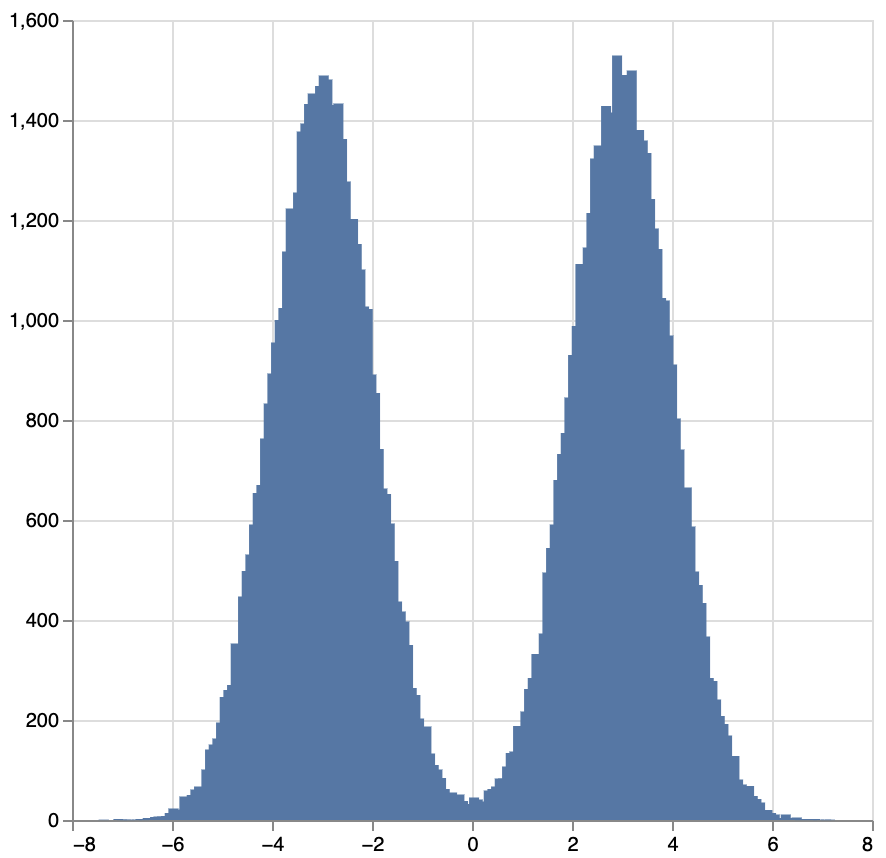Define distributions as programs

Perform inference with a variety of standard methods defined compositionally

Integrate with Haskell code like this because Monad-Bayes is just a library, not a separate language

## Example¶

model :: Distribution Double
model = do
x <- bernoulli 0.5
normal (if x then (-3) else 3) 1

image :: Distribution Plot
image = fmap (plot . histogram 200) (replicateM 100000 model)

sampler image


The program model is a mixture of Gaussians. Its type Distribution Double represents a distribution over reals. image is a program too: as its type shows, it is a distribution over plots. In particular, plots that arise from forming a 200 bin histogram out of 100000 independent identically distributed (iid) draws from model. To sample from image, we simply write sampler image, with the result shown below: